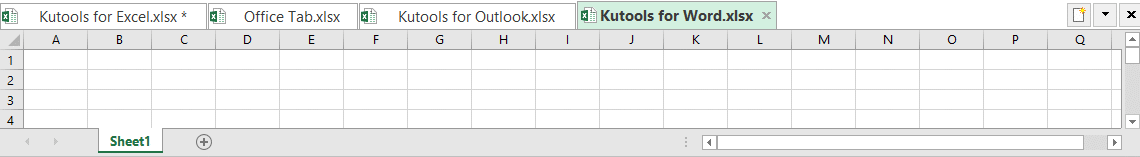Note: The other languages of the website are Google-translated. Back to English

## How to use INDEX and MATCH together in Excel

When working with Excel tables, you may constantly find situations that you need to look up a value. In this tutorial, we will show you how to apply the combination of the INDEX and MATCH functions to make horizontal and vertical lookups, two-way lookups, case-sensitive lookups, and the lookups that meet multiple criteria.

What do INDEX and MATCH functions do in Excel

How to use INDEX and MATCH functions together

#### What do INDEX and MATCH functions do in Excel

Before we use the INDEX and MATCH functions, let’s make sure we know how can INDEX and MATCH help us in looking up values first.

##### Usage of INDEX function in Excel

The INDEX function in Excel returns the value at a given location in a specific range. The syntax of the INDEX function is as follows:

=INDEX(array, row_num, [column_num])
• array (required) refers to the range where you want to return the value from.
• row_num (required, unless column_num is present) refers to the row number of the array.
• column_num (optional, but required if row_num is omitted) refers to the column number of the array.

For example, to know the final exam score of Jeff, the 6th student on the list, you can use the INDEX function like this:

=INDEX(E2:E11, 6) >>> returns 60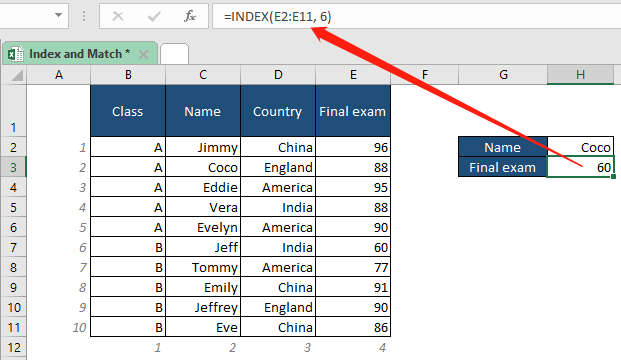√ Note: The range E2:E11 is where the final exam listed, while the number 6 finds the exam score of the 6th student.

Here let’s do a little test. For the formula =INDEX(B2:E2,3), what value will it return? ---Yes, it will return China, the 3rd value in the given range.

Now we should know that the INDEX function can work perfectly with horizontal or vertical ranges. But what if we need it to return a value in a greater range with several rows and columns? Well, in this case, we should apply both a row number and a column number. For example, to find out the country Emily comes from with INDEX, we can locate the value with a row number of 8 and a column number of 3 in the cells through B2 to E11 like this:

=INDEX(B2:E11,8,3) >>> returns China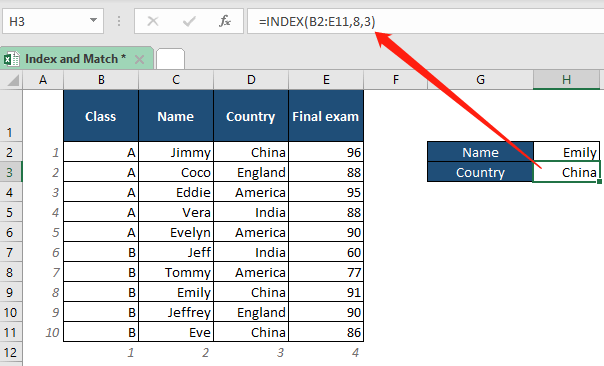According to the examples above, about the INDEX function in Excel, you should know that:

• The INDEX function can work with vertical and horizontal ranges.
• The INDEX function is not case-sensitive.
• The row number goes ahead of the column number (if you need both numbers) in the INDEX formula.

However, for a really big database with multiple rows and columns, it’s certainly not convenient for us to apply the formula with an exact row number and column number. And this it when we should combine the use of the MATCH function.

Now, let’s learn about the basics of the MATCH function first.

##### Usage of MATCH function in Excel

The MATCH function in Excel returns a numeric value, the location of a specific item in the given range. The syntax of the MATCH function is as follows:

=MATCH(lookup_value, lookup_array, [match_type])
• lookup_array (required) refers to the range of cells where you want MATCH to search.
• match_type (optional), 1, 0 or -1:
• 1(default), MATCH will find the largest value that is less than or equal to the lookup_value. The values in the lookup_array must be placed in ascending order.
• 0, MATCH will find the first value that exactly equals the lookup_value. The values in the lookup_array can be in any order. (For the cases that the match type is set to 0, you can use wildcard characters.)
• -1, MATCH will find the smallest value that is greater than or equal to the lookup_value. The values in the lookup_array must be placed in descending order.

For example, to know the position of Vera in the list of names, you can use the MATCH formula like this:

=MATCH("vera",C2:C11,0) >>> returns 4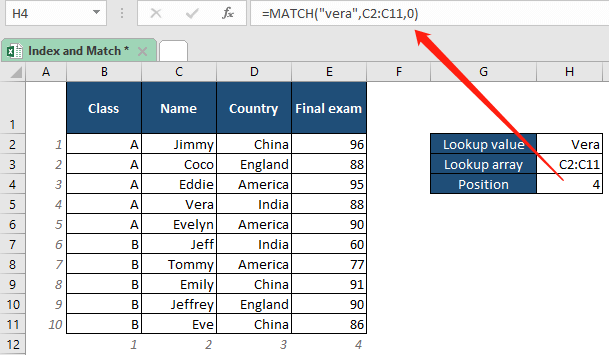√ Note: The MATCH function is not case-sensitive. The result “4” indicates that the name “Vera” is in the 4th position of the list. The “0” in the formula is the match type that will find the first value in the lookup array that equals exactly to the lookup value “Vera”.

To know the position of the score “96” in the row from B2 to E2, you can use MATCH like this:

=MATCH(96,B2:E2,0) >>> returns 4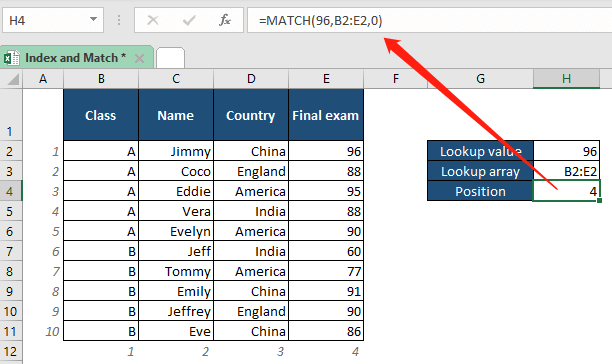☞ Things we should know about the MATCH function in Excel:

• The MATCH function returns the position of the lookup value in the lookup array, not the value itself.
• The MATCH function returns the first match in case of duplicates.
• Just like the INDEX function, the MATCH function can work with vertical and horizontal ranges as well.
• MATCH is not case-sensitive as well.
• If the lookup value of the MATCH formula is in the form of text, enclose it in quotes.

Now that we know about the basic usages of the INDEX and MATCH functions in Excel, let’s roll up our sleeves and get ready to combine the two functions.

#### How to use INDEX and MATCH functions together

In this part, we will talk about different circumstances to use the INDEX and MATCH functions to meet different needs.

##### Example to combine INDEX and MATCH

Please see the example below to figure out how can we combine the INDEX and MATCH functions:

For example, to know Evelyn’s final exam score, we should use the formula:

=INDEX(A2:D11,MATCH("evelyn",B2:B11,0),MATCH("final exam",A1:D1,0)) >>> returns 90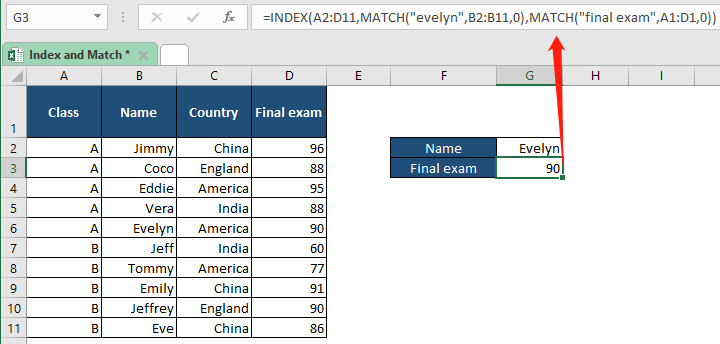Well, since the formula may look complicated, let’s go through each part of it.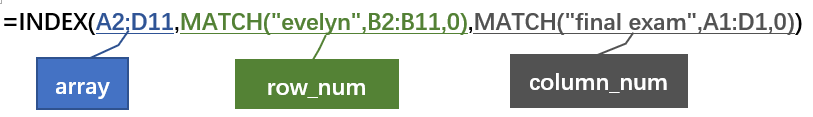As you can see above, the big INDEX formula contains three arguments:

• array: A2:D11 tells INDEX to return the matching value from the cells through A2 to D11.
• row_num: MATCH("evelyn",B2:B11,0) tells INDEX the exact row of the value.
• About the MATCH formula, we can explain it as: to return the position of the first value that is exactly equal to “evelyn” in the cells from B2 to B11 in a numeric value, which is 5.
• column_num: MATCH("final exam",A1:D1,0) tells INDEX the exact column of the value.
• About the MATCH formula, we can explain it as: to return the position of the first value that is exactly equal to “final exam” in the cells from A1 to D1 in a numeric value, which is 4.

So, you can see the big formula as simple as the one we showed below:

=INDEX(A2:D11,5,4)

In the example, we used hardcoded values, "evelyn" and "final exam". However, in such a big formula, we don’t want hardcoded values as we will have to change them each time we are going to search for something new. In such circumstances, we can use cell references to make the formula dynamic like this:

=INDEX(A2:D11,MATCH(G2,B2:B11,0),MATCH(F3,A1:D1,0))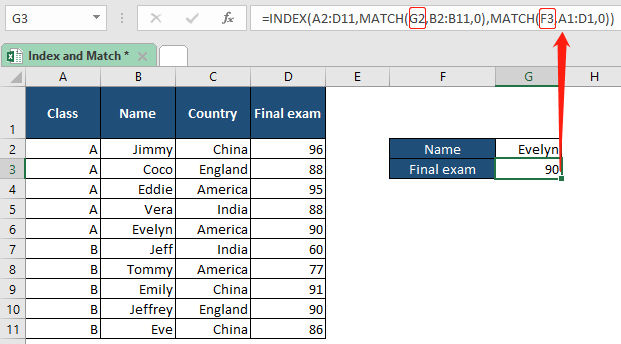##### INDEX and MATCH to apply a left lookup

Now, let’s say you need to know Evelyn’s class, how can we use INDEX and MATCH to know the answer? If you paid attention, you should notice that the class column is at the left side of the name column, and which is beyond the ability of another Excel’s powerful lookup function, the VLOOKUP.

In fact, the left lookup ability happens to be one of the aspects where the combination of INDEX and MATCH is superior to VLOOKUP.

To know Evelyn’s class, all you need to do is to change the value in the cell F3 to “Class”, and use the same formula as shown above, the INDEX and MATCH functions will then tell you the answer right away:

=INDEX(A2:D11,MATCH(G2,B2:B11,0),MATCH(F3,A1:D1,0)) >>> returns A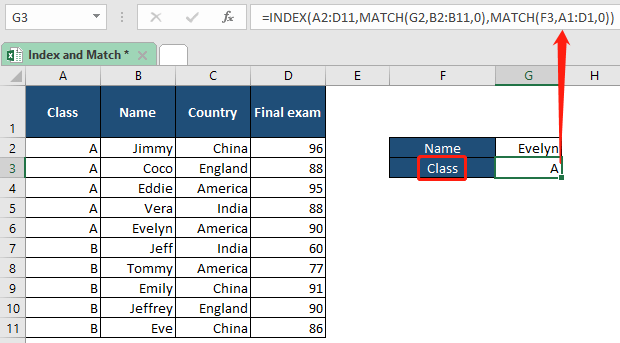If you have instal a professional Excel addin developed by our team, you can also apply a left lookup for specified values with its LOOKUP from Right to Left feature with few clicks. To implement the feature, please go to the Kutools tab in your excel, find the Formula group, and click LOOKUP from Right to Left on the drop-down list of Super LOOKUP. You will see a pop-up dialogue box like this: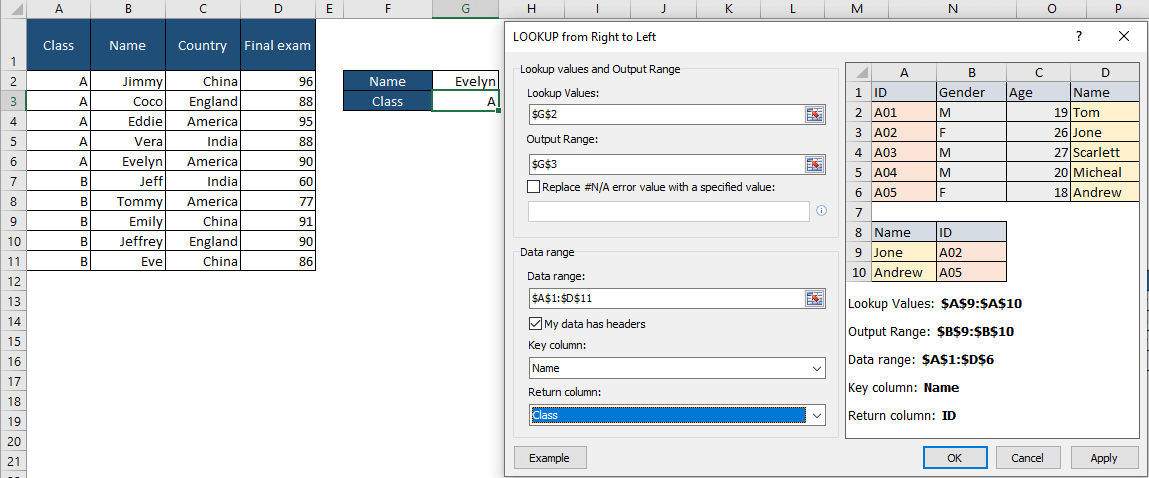Click here for concrete steps to apply the left lookup feature with Kutools for Excel.

##### INDEX and MATCH to apply a two-way lookup

Now, are you able to make the INDEX and MATCH combination formula with dynamic lookup values to apply two-way lookups? Let’s practice making formulas in the cells G3, G4, and G5 as shown below: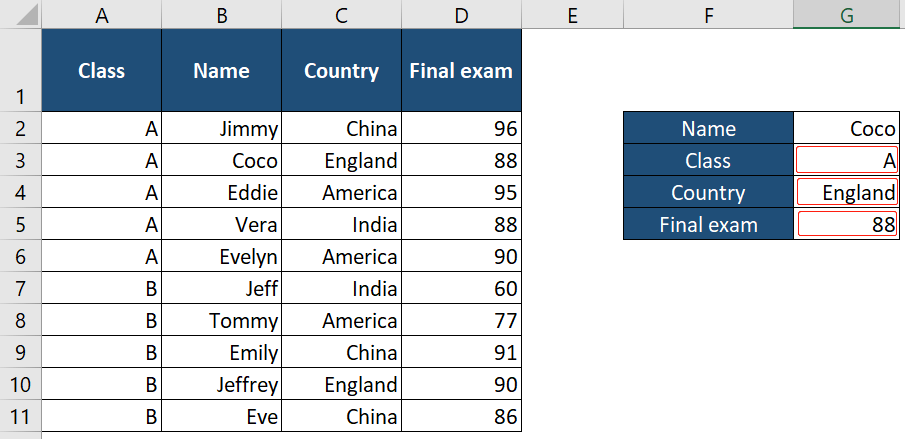Cell G3: =INDEX(A2:D11,MATCH(G2,B2:B11,0),MATCH(F3,A1:D1,0))
Cell G4: =INDEX(A2:D11,MATCH(G2,B2:B11,0),MATCH(F4,A1:D1,0))
Cell G5: =INDEX(A2:D11,MATCH(G2,B2:B11,0),MATCH(F5,A1:D1,0))

√ Note: After applying the formulas, you can easily get the information of any students by changing the name in cell G2.

##### INDEX and MATCH to apply a case-sensitive lookup

From the above examples, we know that the INDEX and MATCH functions are not case-sensitive. However, in the cases you need your formula to distinguish upper-and-lower-case characters, you can add EXACT function to your formulas like this:

=INDEX(return_range,MATCH(TRUE,EXACT("lookup_value1",range1),0),MATCH("lookup_value2",range2,0))
√ Note: This is an array formula that requires you to enter with Ctrl + Shift + Enter. A pair of curly brackets will then show up in the formula bar.

For example, to know JIMMY’s exam score, use the functions like this: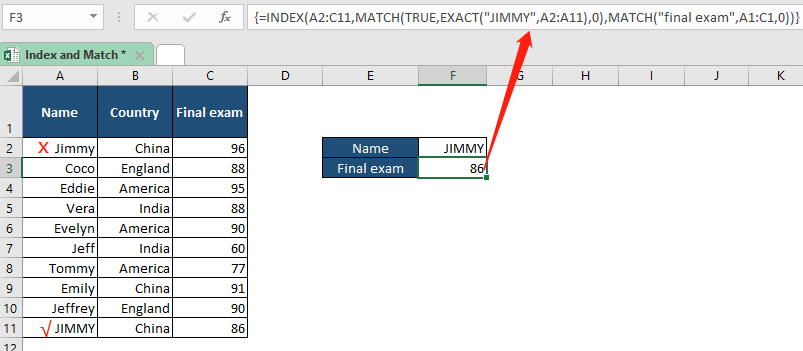=INDEX(A2:C11,MATCH(TRUE,EXACT("JIMMY",A2:A11),0),MATCH("final exam",A1:C1,0)) >>> returns 86

Or you can use cell references:

=INDEX(A2:C11,MATCH(TRUE,EXACT(F2,A2:A11),0),MATCH(E3,A1:C1,0)) >>> returns 86
Note: Don’t forget to enter with Ctrl + Shift + Enter.

##### INDEX and MATCH to apply a lookup with multiple criteria

When dealing with a big database with several columns and row captions, it’s always tricky to find something that meets multiple conditions. In this case, please see the formula below to lookup multiple criteria:

=INDEX(return_range,MATCH(1,(lookup_value1=range1)*(lookup_value2=range2)*(…),0))
Note: This is an array formula that requires you to enter with Ctrl + Shift + Enter. A pair of curly brackets will then show up in the formula bar.

For example, to find the final exam score of Class A’s Coco who is from India, the formula is as follows: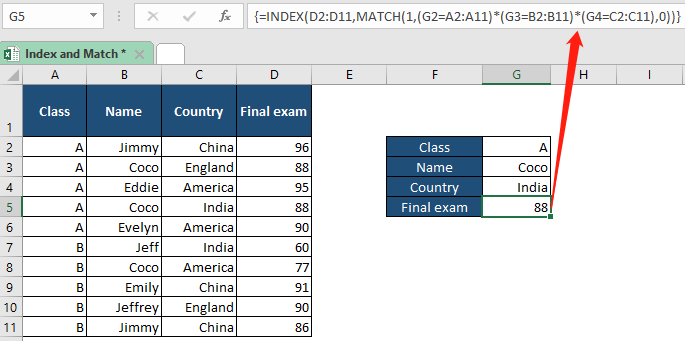=INDEX(D2:D11,MATCH(1,(G2=A2:A11)*(G3=B2:B11)*(G4=C2:C11),0)) >>> returns 88
√ Note: Don’t forget to enter with Ctrl + Shift + Enter.

Well, what if you constantly forget to use Ctrl + Shift + Enter to complete the formula so that the formula returns incorrect results? Here we have a more complex formula, with which you can just complete with one simple Enter key:

=INDEX(return_range,MATCH(1,INDEX((lookup_value1=range1)*(lookup_value2=range2)*(…),0,1),0))

For the same example above to find the final exam score of Class A’s Coco who is from India, the formula that only needs a usual Enter hit is as follows: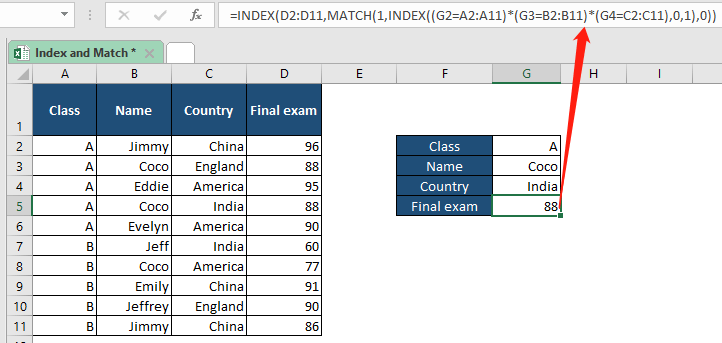=INDEX(D2:D11,MATCH(1,INDEX((G2=A2:A11)*(G3=B2:B11)*(G4=C2:C11),0,1),0)) >>> returns 88

Here we won’t use hardcoded values, as we will want a universal formula in the case with multiple criteria. Only in this way, we can easily get the result we want by changing the values in cells G2, G3, G4 in the example above.

Withs Multi-condition Lookup feature, you can look up specific values with multiple criteria in few clicks. To implement the feature, please go to the Kutools tab in your excel, find the Formula group, and click Multi-condition Lookup on the drop-down list of Super LOOKUP. You will then see a pop-up dialogue box as shown below: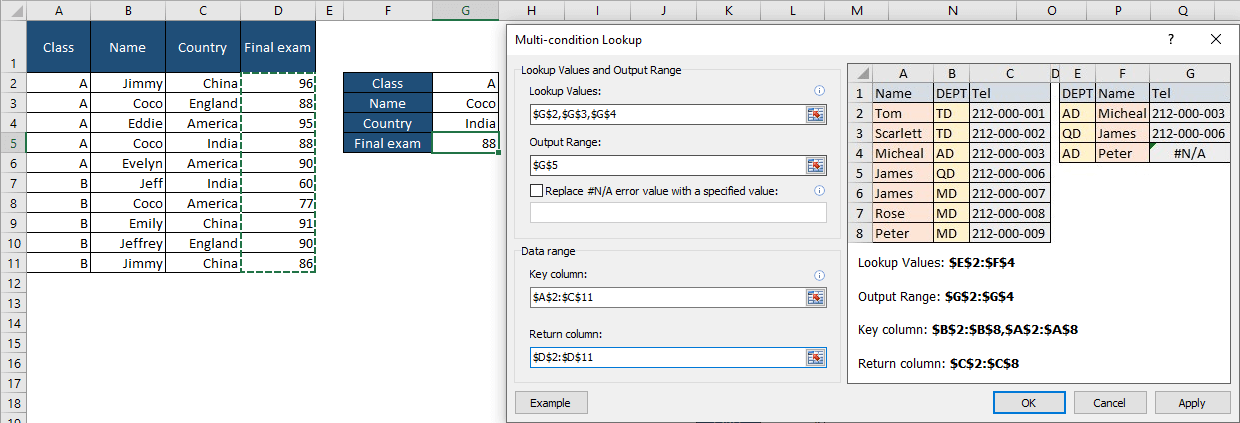Click here for concrete steps to apply the multi-condition lookup feature with Kutools for Excel.

##### INDEX and MATCH to apply a lookup across multiple columns

If we have an Excel spreadsheet with different columns sharing one caption as shown below, how can we match each student’s name with his/her class with INDEX and MATCH?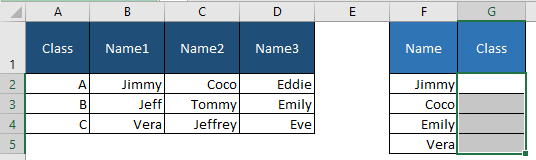Here, let me show you the way to complete the task with our professional tool. With its Formula Helper, you can quickly match students with their classes as the steps shown below:

1. Select the destination cell where you want to apply the function.

2. Under the Kutools tab, go to Formula Helper, click Formula Helper on the drop-down list.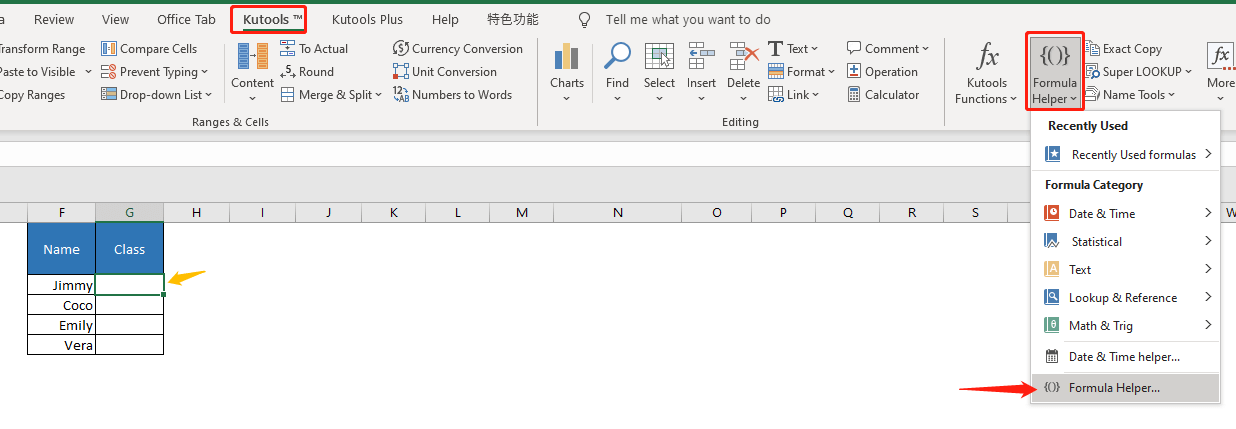3. Choose Lookup from Formula Type, then click on Index and match on multiple columns.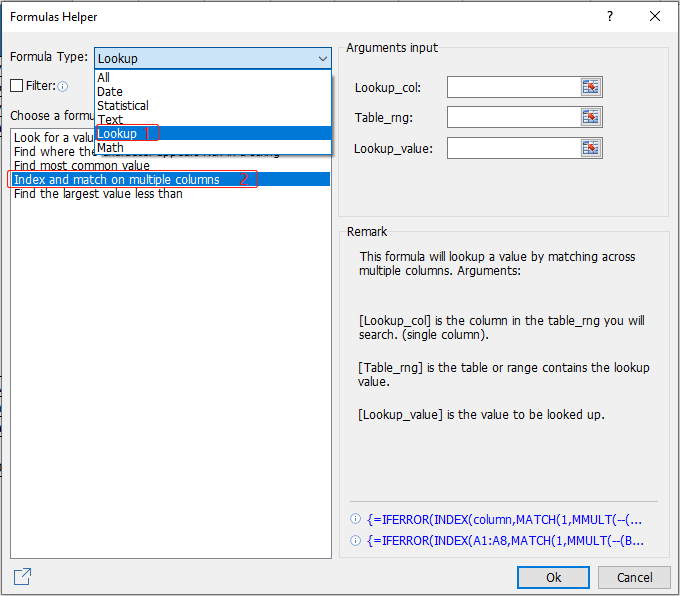4. a. Click the 1st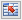button at the right side of Lookup_col to select the cells that you want to return a value from, i.e., the class names. (You can only select a single column or row here.)
b. Click the 2ndbutton at the right side of Table_rng to select the cells to match the values in the selected Lookup_col, i.e., the students’ names.
c. Click the 3rdbutton at the right side of Lookup_value to select the cell to be looked up, i.e., the student’s name that you want to match with his/her class.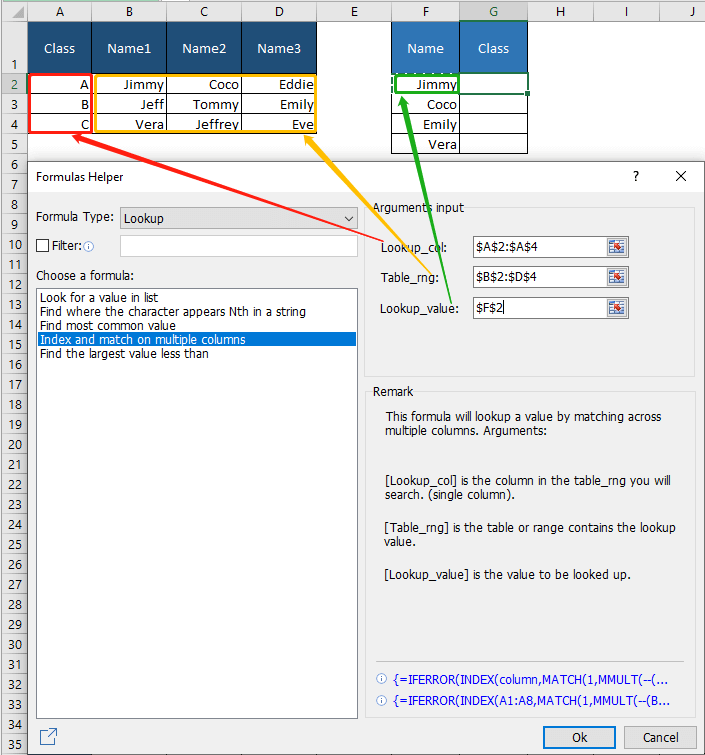5. Click Ok, you will see the class name of Jimmy showed up in the destination cell.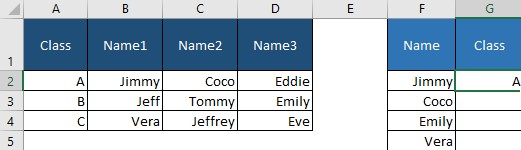6. Now you can drag the fill handle down to fill in other students’ classes.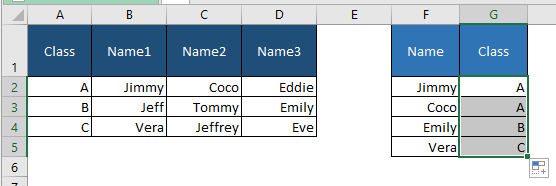## The Best Office Productivity Tools

### Kutools for Excel Solves Most of Your Problems, and Increases Your Productivity by 80%

• Super Formula Bar (easily edit multiple lines of text and formula); Reading Layout (easily read and edit large numbers of cells); Paste to Filtered Range...
• Merge Cells/Rows/Columns and Keeping Data; Split Cells Content; Combine Duplicate Rows and Sum/Average... Prevent Duplicate Cells; Compare Ranges...
• Select Duplicate or Unique Rows; Select Blank Rows (all cells are empty); Super Find and Fuzzy Find in Many Workbooks; Random Select...
• Exact Copy Multiple Cells without changing formula reference; Auto Create References to Multiple Sheets; Insert Bullets, Check Boxes and more...
• Favorite and Quickly Insert Formulas, Ranges, Charts and Pictures; Encrypt Cells with password; Create Mailing List and send emails...
• Extract Text, Add Text, Remove by Position, Remove Space; Create and Print Paging Subtotals; Convert Between Cells Content and Comments...
• Super Filter (save and apply filter schemes to other sheets); Advanced Sort by month/week/day, frequency and more; Special Filter by bold, italic...
• Combine Workbooks and WorkSheets; Merge Tables based on key columns; Split Data into Multiple Sheets; Batch Convert xls, xlsx and PDF...
• Pivot Table Grouping by week number, day of week and more... Show Unlocked, Locked Cells by different colors; Highlight Cells That Have Formula/Name...### Office Tab - brings tabbed interface to Office, and make your work much easier

• Enable tabbed editing and reading in Word, Excel, PowerPoint, Publisher, Access, Visio and Project.
• Open and create multiple documents in new tabs of the same window, rather than in new windows.
• Increases your productivity by 50%, and reduces hundreds of mouse clicks for you every day!